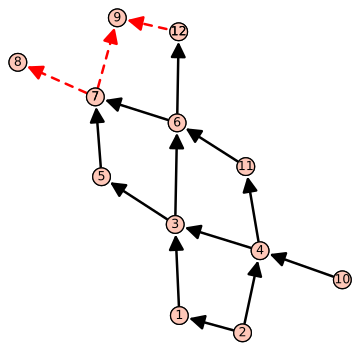# Drawing Auslander-Reiten quivers with sage possible?

Hi,

I'd like to draw an Auslander-Reiten quiver with sage. I tried to do this by viewing the Auslander-Reiten quiver as a digraph, but, unfortunately, I was not able to find out how to let sage draw both dashed and solid arrows in one digraph.

Is this possible? If not, is there another way to let sage draw Auslander-Reiten quivers?

Any help would be appreciated.

Edit:

I used the following code:

sage: H=DiGraph()

After having typed

sage: H.show()

a few times I got the following output:Now, I'd like to draw dahed arrows indicating the Auslander-Reiten translates (e.g. a dashed arrow from 3 to 2).

edit retag close merge delete

Please provide the code you used in your attempts so far. It might be a good starting point for someone to help.

Thank you very much for your comment. I edited the question.

1

Note that vertices of a graph can also be pairs (i,j), that could be very useful here. You can also choose the positions of the vertices using a dictionary. Read the doc: DiGraph?

@Bern, maybe you can also say how you produce your Auslander-Reiten quiver? And how you use that quiver to choose the edges of your digraph.

It seems like the edge_style attribute for graph plots applies to all edges of a graph.

Sort by » oldest newest most votedFollowing the suggestion from @FrédéricC in the comments.

Here is a bit of code that might help.

def draw_quiver(E1,E2):
H = DiGraph(E1+E2)
H.layout('spring',save_pos=True)
H1=DiGraph(solid_edges)
P1=H1.plot(pos=H.get_pos())
H2=DiGraph(dashed_edges)
P2=H2.plot(pos=H.get_pos(),edge_colors={'red':dashed_edges},edge_style='--')
return P1+P2


Then the following code

solid_edges = [(2,1),(2,4),(10,4),(1,3),(4,3),(4,11),(11,6),(3,6),(3,5),(5,7),(6,7),(6,12)]
dashed_edges = [(7,8),(7,9),(12,9)]
draw_quiver(solid_edges,dashed_edges).show(axes=False)


produces the png displayed below.more

Thank you very much for your answer (and sorry for my very late reply). It works perfectly fine! :-)